# SAT II Math II : Maximum and Minimum

## Example Questions

### Example Question #42 : Properties Of Functions And Graphs

What is the vertex of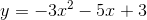?  Is it a max or min?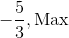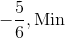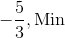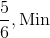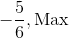Explanation:

The polynomial is in standard form of a parabola.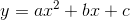To determine the vertex, first write the formula.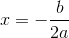Substitute the coefficients.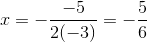Since theis negative is negative, the parabola opens down, and we will have a maximum.

The answer is:### Example Question #43 : Properties Of Functions And Graphs

Given the parabola equation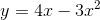, what is the max or minimum, and where?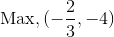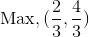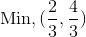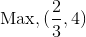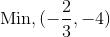Explanation:

The parabola is in the form: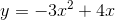The vertex formula will determine the x-value of the max or min.  Since the value ofis negative, the parabola will open downward, and there will be a maximum.

Write the vertex formula and substitute the correct coefficients.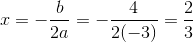Substitute this value back in the parabolic equation to determine the y-value.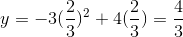The answer is: